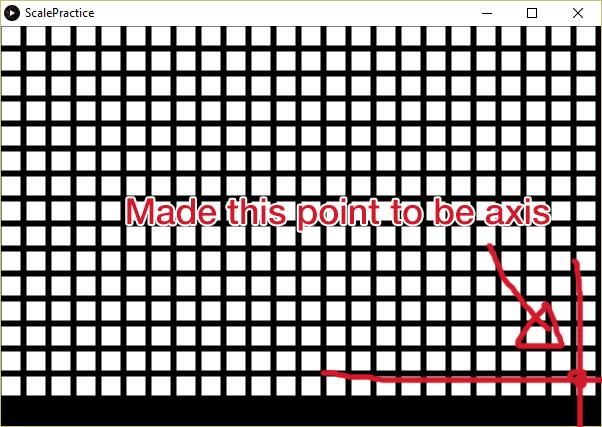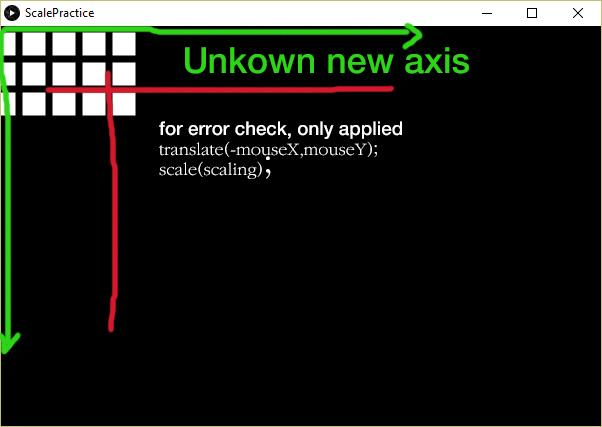#### Howdy, Stranger!

We are about to switch to a new forum software. Until then we have removed the registration on this forum.

# Problem with 2D transformation..

edited January 2016

Hello, I'm trying to implement screen zooming in/out by axis from mouse position. My very first idea was this:

``````    translate(-mouseX,-mouseY);
scale(scaling);
translate(mouseX,mouseY);
``````

Here is my code:

``````//Code Starts
void settings(){
size(600,400,OPENGL);
}

void setup(){
background(0);

scaleLevel=1;
xAxis=0;
yAxis=0;
}

void draw(){
background(0);
transform();
drawRects();
}

void drawRects(){
for(int i=0; i<24;i++){
for(int j=0; j<15; j++){
fill(255);
rectMode(LEFT);
rect(i*25,j*25,i*25+20,j*25+20);
}
}
}

void mouseWheel(MouseEvent event) {

//Update Scale Axis
xAxis = mouseX;
yAxis = mouseY;

//This method controls scaleLevel variable
if(event.getCount()==-1)
scaleLevel += 0.2;
else if(event.getCount()==1)
if(scaleLevel>=1.2)
scaleLevel -= 0.2;
else
scaleLevel = 1;

System.out.println("Heatmap>> Scale Level: "+scaleLevel);
}

void transform(){
translate(-1*xAxis,-1*yAxis);
scale(scaleLevel);
}

float scaleLevel;
int xAxis;
int yAxis;
``````

However, when I tested this, the axis seemed to move little bit more forward, which mouseX,Y wasn't the axis. sperate transformation worked fine, but when I come all three together, the result goes unpredictable.Tagged:

• Post a working sketch. It's much easier for someone to help you if they have something they can copy, paste, run, see your problem, and fix.

To help you right now, I would need to start by trying to replicate the work you have already done, replicate your problems, and then solve it. That's a lot more work!

• ``````float angleBox1; // angle 1

float scaleLevel = 1.0; // scale

float offsetXToUse; // translate data to use
float offsetYToUse; // use those for translate

float offsetX; // translate data internally
float offsetY; // don't use those for translate

// ----------------------------------------------

void setup() {
size(900, 990, OPENGL);
background(0);
} // func

void draw() {
background(0);
lights();

camera(0, 0, (height/2.0) / tan(PI*30.0 / 180.0),
0, 0, 0,
0, 1, 0);

pushMatrix();
translate(offsetXToUse, offsetYToUse);
scale (scaleLevel);
// draw stuff
drawScene();
popMatrix();

// HUD
camera();
noLights();
fill(255);
text("move mouse to translate, use mouse wheel to zoom",
20, 20);
} // func

// -----------------------------------------------
// Inputs

void mouseWheel(MouseEvent event) {

//This method controls scaleLevel variable

if (event.getCount()==-1)
scaleLevel += 0.2;
else if (event.getCount()==1)
if (scaleLevel>=.3)
scaleLevel -= 0.3;
else
scaleLevel = .3;

// check lower boundary
if (scaleLevel<.1)
scaleLevel = .1;
println("Scale Level: "+scaleLevel);
}

void mouseMoved() {

//This method controls offsetXToUse and offsetYToUse variable

offsetX += mouseX-pmouseX;
offsetY += mouseY-pmouseY;

offsetXToUse = map(offsetX, 0, width, -width/2, width/2); // translate data to use
offsetYToUse = map(offsetY, 0, height, -height/2, height/2);
}

// --------------------------------------------
// other functions

void drawScene() {
pushMatrix();
fill(111, 111, 222);
translate(0, 8, 0);
rotateY(angleBox1);
// noFill();
// stroke(255);
noStroke();
box(40);
popMatrix();

pushMatrix();
translate(-58, -48, -200);
fill(255, 0, 0);
noStroke();
sphere (30);
popMatrix();

pushMatrix();
fill(111);
translate(48, 248, 0);
rotateY(.5);
//  noFill();
stroke(255);
box(40);
popMatrix();

angleBox1+=.03;
}
//
``````
• of course you can also apply the mouse movement to rotation:

``````// version with rotation und zoom

float angleBox1; // angle 1

float scaleLevel = 1.0; // scale

float rotateXToUse; // rotate data to use (in radians)
float rotateYToUse; // use those for rotate

float rotateX; // rotate data internally
float rotateY; // don't use those for rotate

// ----------------------------------------------

void setup() {
size(900, 990, OPENGL);
background(0);
} // func

void draw() {
background(0);
lights();

camera(0, 0, (height/2.0) / tan(PI*30.0 / 180.0),
0, 0, 0,
0, 1, 0);

pushMatrix();
rotateY(rotateXToUse);
rotateX(rotateYToUse);
scale (scaleLevel);
// draw stuff
drawScene();
popMatrix();

// HUD
camera();
noLights();
fill(255);
text("move mouse to ROTATE (space bar: reset rotation), use mouse wheel to zoom",
20, 20);
} // func

// -----------------------------------------------
// Inputs

void mouseWheel(MouseEvent event) {

//This method controls scaleLevel variable

if (event.getCount()==-1)
scaleLevel += 0.2;
else if (event.getCount()==1)
if (scaleLevel>=.3)
scaleLevel -= 0.3;
else
scaleLevel = .3;

// check lower boundary
if (scaleLevel<.1)
scaleLevel = .1;
println("Scale Level: "+scaleLevel);
}

void mouseMoved() {

//This method controls rotateXToUse and rotateYToUse variable

rotateX += mouseX-pmouseX; // internal rotate data
rotateY += mouseY-pmouseY;

rotateXToUse = (map(rotateX, 0, width, -PI, PI)); // rotate data to use
rotateYToUse = (map(rotateY, 0, height, PI, -PI));
}

void keyPressed() {
rotateXToUse = 0.0;
rotateYToUse = 0.0;
}

// --------------------------------------------
// other functions

void drawScene() {
pushMatrix();
fill(111, 111, 222);
translate(0, 8, 0);
rotateY(angleBox1);
// noFill();
// stroke(255);
noStroke();
box(40);
popMatrix();

pushMatrix();
translate(-58, -48, -200);
fill(255, 0, 0);
noStroke();
sphere (30);
popMatrix();

pushMatrix();
fill(111);
translate(48, 248, 0);
rotateY(.5);
//  noFill();
stroke(255);
box(40);
popMatrix();

angleBox1+=.03;
}
//
``````
• how do you show your code like that? when i copy and paste my code in, it looks very messy were yours is neat??

• Magic.

• haha no seriously tho :( i have a question about my snake game as im new to this and i cant get my code to preview like this

• thank you

• darn.

;-)